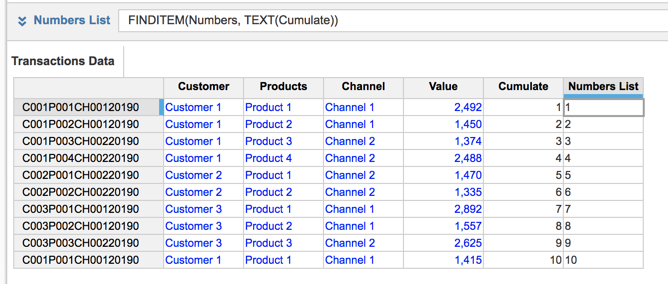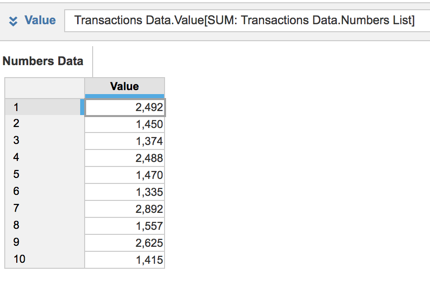# sequential numbering in line itemHi,

I would like to know how can we create a sequential numbering in a line item. I saw some post where they use RANK but it does not fit the requirement needed.

In the module attached, I have a line item Transaction number where I want to show automatic numbering (1 - 100 for example) of the number of transactions. This will be used once it is imported to another model where there is a numbered list present. Is there a formula that will automatically show the numbers on the line item.

Regards,

Tagged:

•Hi Jsdeloria21,

If this is a numbered list, then it should be straight forward to use the number of the numbered list and generate a unique number.

If this is a normal list, I would suggest using Rank Formula to compute the transaction number. PFB the screenshots that would suffice the requirement.

1. Use the below formula to rank the transactions accordingly

RANK(1, ASCENDING, SEQUENTIAL)I am not sure about your challenge of using rank, so if you can more elaborate.

Another solution can be we use the numbers of the Transaction IDs (Using Right/Left) and truncate the unique numbers from them if its not a numbered list.

Thanks and Regards,

Akhil

•You can also CUMULATE on a list (although this is dependant on the order in which the lists members are created)

The formula is CUMULATE(1, FALSE, list name), the FALSE

In your transaction list, ensure that the code is set 1,2,3 etc and then you can use FINDITEM(Transaction list,TEXT(Cumulate line item)) to return the Transaction id against the source list

You can then use a SUM formula to pull the figures though into the target module

In my example below, I have a 'Transactions' list that I want to match to my 'Numbers' listI hope this helps

David